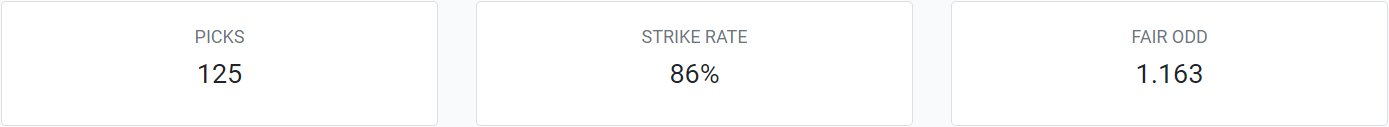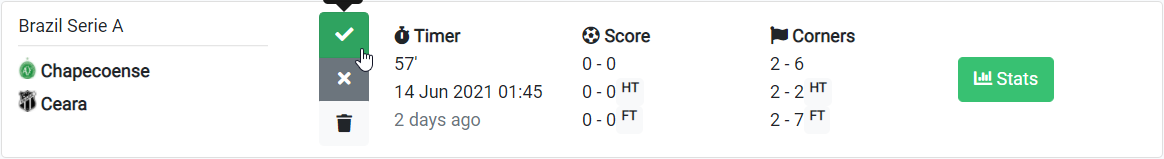The Strike Rate is a quick indicator of strategy performance as it represents the percentage of matches are being correctly picked by your strategy.The Strike Rate is calculated based on the matches that you have marked as a Hit [✔️] out of all the matches that you marked. Unmarked or deleted matches don't count towards the strike rate. (e.g. if there are 50 picked matches in total, 10 are a Miss, and 30 are a Hit, the strike rate is 75%, i.e. 30 out of 40).The Fair odd is simply the decimal odd value that corresponds to the strike rate percentage (e.g a Strike Rate of 50% = Fair Odd of 2.00) (https://inplayguru.com/tools/odds-converter). Notice that this value becomes more statistically relevant as the sample size grows.